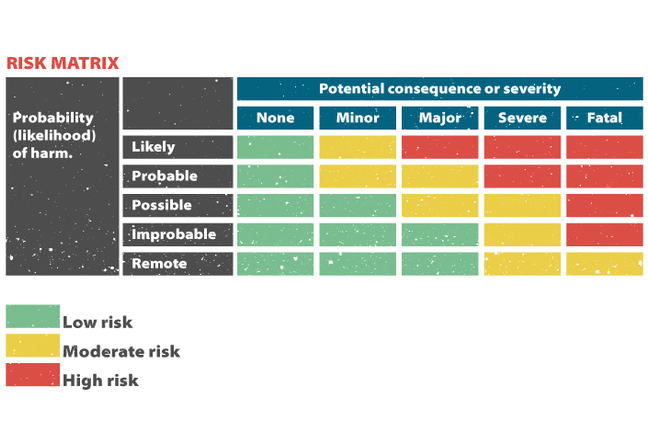1.11

## University of BergenRisk matrix illustrating the risk is a function of the probability that a harmful event will occur and the consequence of this event.

# Risk matrix

In any risk assessment, the degree of risk and thus prioritization for action is, to a certain extent, based on personal judgement and opinion. Although it is recommended to “keep it simple”, prioritisation of specific actions should be based on sound reasons.

A risk matrix illustrates the principles of risk evaluation that could be used to make decisions on how to prioritize control measures. In this matrix the likelihood of harm and the potential severity of that harm could be categorized as indicated in the figure below. The risk level (red, yellow and green areas) determines which risks should be tackled first, starting with the highest risks (red area). When you evaluate the level of a risk you should pay attention to a number of things.

1: What is the likelihood/probability of the exposure to the hazard.

2: What is the consequence of exposure to the hazard.

3: Using the two variable risk matrix, determine the risk rating from the likelihood and consequence descriptors.

In the tiger example the likelihood/probability of the harm would be remote when the tiger is in the cage even though the potential severity/consequence of the harm would be fatal, - thus the risk is moderate (yellow area). If out of the cage, the probability of harm would be likely, the consequence fatal, and the risk high (red area).

Analogously, in the case of miners in the risk assessment example described in the previous step, the potential health consequence associated with exposure to crystalline silica over some years is severe since it may cause irreversible and disabling lung disease (silicosis). When drilling, the probability is likely that exposure levels exceed the occupational exposure limits (OEL). According to the risk matrix (figure above), the health risk will be high (red zone; likely probability of harmful exposure and severe consequence) when no precautions have been taken. But when reducing exposure by use of dust suppressive techniques, the probability of high exposure compared to OEL is reduced from likely to possible, and the resulting health risk is reduced from high to moderate (yellow zone). This is an example of a risk reducing measure.

There are several options for how to design a risk matrix, and for creating scales for probability of harm and potential severity as well as for categorization of risk levels. The discussion here was just an example of how to compare risks associated with exposure to different hazards, in order to prioritize control measures.

A methodology for risk assessment using a risk matrix is described by the The University of Melbourne